# The Logic of Complex Predicates Examples

For convenience, we reproduce the item Logic/Logic of Complex Predicates from Principia Metaphysica: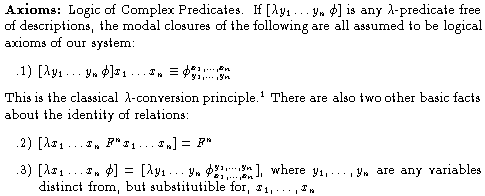In this tutorial, we give examples of the axioms and then draw out some consequences.

## Examples of the Axioms

Instances of Axiom 1:The first example asserts: objects c and a exemplify being an x and y such that x, b, and y exemplify S if and only if c, b, and a exemplify S. As an intuitive example, we might say: John and Betty stand in the relation being an x and y such that x gives Fido to y if and only if John gives Fido to Betty. The second example asserts: objects b and a exemplify being an x and y such that necessarily, x fails to bear P to y if and only if necessarily, b fails to bear P to a. As a more concrete example: Sherlock Holmes and Gladstone exemplify being an x and y such that necessarily x and fail to meet each other if and only if necessarily, Holmes fails to meet Gladstone (the right condition in this biconditional is in fact true---an abstract object like Holmes is not the kind of thing that could meet an ordinary object such as Gladstone, and so given this principle, these two objects exemplify the complex relation denoted by the complex predicate; note, however, that the right condition of the biconditional is consistent with the claim: according to the Conan Doyle novels, Holmes met Gladstone). The third example asserts: object b exemplifies being an object x such that, necessarily, if x exemplifies Q, then x exemplifies having a spatiotemporal location if and only if necessarily, if b exemplifies Q, then b has a spatiotemporal location. As a concrete instance: an object y exemplifies being something such that, necessarily, if it exemplifies being a plant, it has a spatiotemporal location iff necessarily, if y exemplifies being a plant, y has a spatiotemporal location (the right condition of this biconditional is true for every object y whatsoever). Exercise: Produce specific examples of the other instances of Axiom 1. Remark: Notice that in the last instance of Axiom 1, we have an example of a 3-place relation that is exemplified just in case a 2-place relation is exemplified. The objects a, b, and c exemplify the relation being an x, y, and z such that x bears R to y just in case a bears R to b. Such relations will play an interesting role in developing the theory of possible worlds. In particular, we will focus on 1-place properties that objects exemplify just in case a proposition (0-place relation) is true. For example, by Axiom 1, we know that an object x exemplifies the property being such that Clinton is President if and only if Clinton is President. By Universal Generalization, it follows that every object x exemplifies being such that Clinton is President if and only if Clinton is President. And by the Rule of Necessitation, it follows that necessarily, every object x exemplifies being such that Clinton is President if and only if Clinton is President. We call these properties that objects exemplify whenever a proposition is true `propositional properties'. For a more precise definition, see the item Logic/Propositional Properties.

Instance of Axiom 2: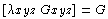This instance asserts: the 3-place relation being an x, y, and z such that x, y, and z exemplify the relation G is identical to the 3-place relation G. In other words, the simplest possible complex predicates, consisting of an atomic exemplification formula in which all the object terms are variables bound by a lambda, denote the same relation as the simple predicate symbol involved in the atomic formula.

Instance of Axiom 3:The first example asserts: the relation being an x and y such that necessarily, it is not the case that x bears P to y is identical with the relation being a y and z such that necessarily, it is not the case that y bears P to z. In other words, interchange of bound variable makes no difference to the relation denoted by the complex predicate.

## Some Consequences:

Logical Theorem: Comprehension Principle for Relations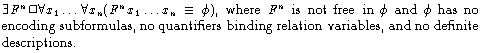This tells us that for any exemplification-based formula phi (containing no quantifiers over relations or definite descriptions), there is a relation F which is necessarily such that objects x_1,...,x_n exemplify F if and only if x_1,...,x_n are such that phi. This is a logical theorem schema that can be derived from Axiom 1 in n+2 simple steps: apply Universal Generalization to Axiom 1 n times, beginning with the variable x_n and ending with the variable x_1; then apply the Rule of Necessitation; finally apply the rule of Existential Generalization to the complex predicate. EG can be applied to the complex predicate because the latter is an n-place term that denotes a relation.

Here are some examples of this logical theorem schema---these correspond to the examples of Axiom 1 above:In each example, the existence of the complex relation in question is explicitly asserted. It is important to recall now that there is a definition of identity for relations. In the section Language/Relation Identity in Principia Metaphysica, one finds the following two definitions, the first of which gives conditions under which properties (1-place relations) are identical and the second of which gives conditions under which n-place relations (n > 1) are identical: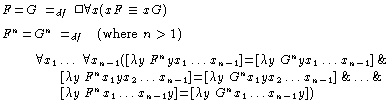The first definition tells us that properties F and G are identical if and only if, necessarily, they encode the same properties. The second definition tells us that, for n > 1, n-place relations F and G are identical iff for each way of plugging n - 1 objects in the same order into F and G, the resulting 1-place properties are identical. These definitions, together with the comprehension principle for relations, constitute a mathematically precise theory of relations and properties, for they are explicit existence and identity conditions for these entities.

Notice that these definitions allow us to consistently assert that there are properties and relations which are necessarily exemplified by the same objects but which are nevertheless distinct. For example, the properties being equiangular and being equilateral are distinct properties, and so it is natural to suppose, therefore, that the property being an equiangular Euclidean triangle is distinct from the property being an equilateral Euclidean triangle. However, these two complex properties are exemplified by the same objects at every possible world (it is necessarily the case that anything exemplifying the one exemplifies the other). This is a virtue of the theory---many theories and/or treatments of properties identify properties that are necessarily equivalent. We identify properties only when they are necessarily equivalent with respect to the objects that encode them, not when they are necessarily equivalent with respect to the objects that exemplify them. The intuition here is that abstract objects represent possible objects of thought. If properties F and G are distinct, then it is possible to conceive of an object having (i.e., encoding) F and not G (and vice versa). So the `encoding extensions' of F and G are distinct when F and G are distinct. However, if F and G are not distinct properties, one couldn't conceive of an object having (i.e., encoding) F and not G. The encoding extensions of identical properties are the same.

Logical (Axioms and) Theorem: Comprehension Principle for PropositionsThis is actually the `degenerate' case of Axiom 1 when n = 0. It can be read as follows: the proposition that-phi is true if and only if phi. Here are some examples:We may read the first example as follows: the proposition that a exemplifies P and it is not the case that b exemplifies Q is true if and only if a exemplifies P and it is not the case that b exemplifies Q.

From the Axiom Schema, we may derive the following theorem schema that constitutes a comprehension principle for propositions:Here are two instances of the theorem schema that correspond to the two instances (above) of the axiom schema: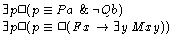These examples explicitly assert the existence of complex propositions.

Here, too, it is important to recall the definition for the identity of propostions in the item Language/Relation Identity:This tells us that propositions p and q are identical if and only if the properties being such that p and being such that q are identical. The conditions under which properties are identical has already been defined, and so propositional identity is hereby reduced to property identity. Consequently, we now have a precise theory of propositions: the comprehension axiom and the above definition give us explicit existence and identity conditions for propositions.

This definition also allows us to consistently assert that certain necessarily equivalent propositions are nevertheless distinct. For example, both of the following propositions are necessarily false: Fido is a dog and it is not the case that Fido is a dog and There is a barber who shaves only those people who don't shave themselves. In formal terms, these propositions would be denoted by the following complex 0-place predicates:Though the two propositions are necessarily equivalent (i.e., true in the same possible worlds), we may consistently assert that they are distinct. This stands in contrast to treatments of propositions on which necessarily equivalent propositions are identified, contrary to intuition.